Test: Dimensional Geometry - 4

# Test: Dimensional Geometry - 4

Test Description

## 20 Questions MCQ Test Topic-wise Tests & Solved Examples for IIT JAM Mathematics | Test: Dimensional Geometry - 4

Test: Dimensional Geometry - 4 for Mathematics 2023 is part of Topic-wise Tests & Solved Examples for IIT JAM Mathematics preparation. The Test: Dimensional Geometry - 4 questions and answers have been prepared according to the Mathematics exam syllabus.The Test: Dimensional Geometry - 4 MCQs are made for Mathematics 2023 Exam. Find important definitions, questions, notes, meanings, examples, exercises, MCQs and online tests for Test: Dimensional Geometry - 4 below.
Solutions of Test: Dimensional Geometry - 4 questions in English are available as part of our Topic-wise Tests & Solved Examples for IIT JAM Mathematics for Mathematics & Test: Dimensional Geometry - 4 solutions in Hindi for Topic-wise Tests & Solved Examples for IIT JAM Mathematics course. Download more important topics, notes, lectures and mock test series for Mathematics Exam by signing up for free. Attempt Test: Dimensional Geometry - 4 | 20 questions in 60 minutes | Mock test for Mathematics preparation | Free important questions MCQ to study Topic-wise Tests & Solved Examples for IIT JAM Mathematics for Mathematics Exam | Download free PDF with solutions
 1 Crore+ students have signed up on EduRev. Have you?
Test: Dimensional Geometry - 4 - Question 1

### The equation of a straight line which makes an angle of 60° with x-axis and passes through the point (√3, 2) is given by

Detailed Solution for Test: Dimensional Geometry - 4 - Question 1

The required equation
(i) makes an angle of 60° with x-axis
(ii) passes through the point (√3 , 2)
Now
(i) ⇒ equation of the straight line will be
y = tan 60° x + c
or y = √3 x + c ...(i)
(ii) ⇒ the coordinates of the point will satisfy equation (i)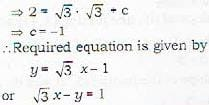Test: Dimensional Geometry - 4 - Question 2

### The line y - x + 2 = 0 cuts the line joining (3, -1) and (8, 9) in the ratio

Detailed Solution for Test: Dimensional Geometry - 4 - Question 2

First remember the following points:
l.The equation of a line passing through two p o in ts (x1,y1) and (x2, y2) is given by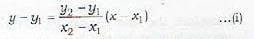2. The. coordinates of the point R which divides the line joining P(x1,y1) and Q(x2, y2) internally in the ratio m1 : m2 are given by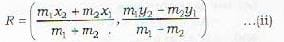3. If R divides PQ externally in the ratio m1 : m2, then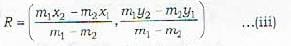Now the given straight line is
y - x + 2 - 0 ...(iv)
The equation of the straight line joining the points (3,-1) and (8, 9) is given by (see (i))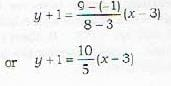or y - 2x + 7 = 0 ...(v)
The point of intersection of (iv) and (v) is obtained on solving these equations and is given by (5,3)
Let the points of intersection (5, 3) divide the line joining (3. -4) and (8. 9) in the ratio m : n.
Then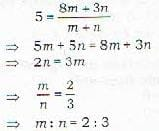Test: Dimensional Geometry - 4 - Question 3

### The equation of the straight line passing through (4, 5) and parallel to the line 2x - 3y = 5 is given by

Detailed Solution for Test: Dimensional Geometry - 4 - Question 3

The given straight line is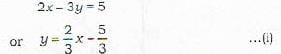The equation of a straight line parallel to (i) will be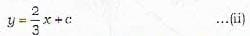Since (ii) passes through P(4, 5), therefore the coordinates of P A\*ill satisfy equation (ii)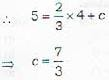∴ Required equation of the straight line is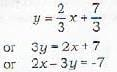Test: Dimensional Geometry - 4 - Question 4

The condition that the three straight lines
ax + by+ c = 0
a1x + b1y + c = 0
a2x + b2y + c2 = 0
may meet in a point, is that, the value of the determinant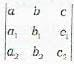must be

Detailed Solution for Test: Dimensional Geometry - 4 - Question 4

The condition for three lines lines to be concurrent is that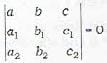Test: Dimensional Geometry - 4 - Question 5

The point (x1,y1) lies on the positive side of the straight line Ax + By + C = 0

Detailed Solution for Test: Dimensional Geometry - 4 - Question 5

First of all learn the following:
Division of a plane by the line
L : Ax + By + C = 0
As shown in the adjoining Figure, the line L divides the plane into three parts, namely I, L and II, where
Ax1 + By1 + C > 0 for all poins (x1, y1) in l
Ax2 + By2 + C = 0 for all points (x2, y2) on L
Ax3 + By3 + C < 0 for all points (x3, y3) in II

Test: Dimensional Geometry - 4 - Question 6

The point (3, 2) lies on the

Detailed Solution for Test: Dimensional Geometry - 4 - Question 6

Given point is (3, 2)
Given straight lines are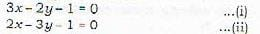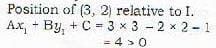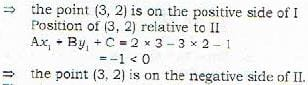Test: Dimensional Geometry - 4 - Question 7

When two straight lines 2x- 3y + 1 = 0 and 3x -6y + 2 = 0 are traced, we get four different compartments. Which of the following four points lie in the same compartment? A(0, 0), B(-1, 1), C(-7, -4), D(9, 6)

Detailed Solution for Test: Dimensional Geometry - 4 - Question 7

The given straight lines are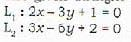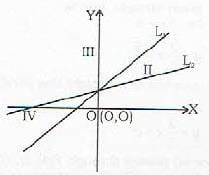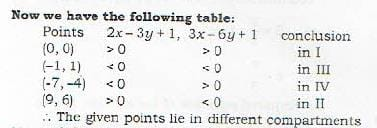Test: Dimensional Geometry - 4 - Question 8

The angle between the lines ax + by + c = 0 and a'x  + b'x + c' = 0 is given by

Detailed Solution for Test: Dimensional Geometry - 4 - Question 8

Comments. The angle φ between two straight lines
y = mx + c and y = m'x + c‘
is given by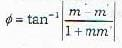Since the straight lines are ax + by + c = 0 and a'x +b'y + c' = 0
therefore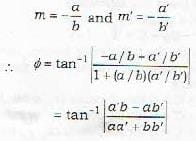Both φ and π - φ shall be the angles between the given lines.

Test: Dimensional Geometry - 4 - Question 9

The straight lines ax + by + c = 0 and a'x + b'y + c' = 0 arc perpendicular if

Detailed Solution for Test: Dimensional Geometry - 4 - Question 9

The angle φ between the lines is given by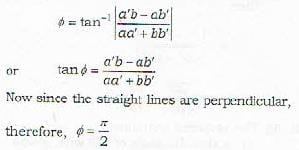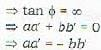Test: Dimensional Geometry - 4 - Question 10

The line passing through the origin is given by

Detailed Solution for Test: Dimensional Geometry - 4 - Question 10

Remark: A straight line passing through the origin shall be of the form
y = mx
and there will not bo any constant term in the eouation.

Test: Dimensional Geometry - 4 - Question 11

The four points (0, 4, 1), (2, 3, -1), (4, 5, 0). (2, 6, 2) are the vertices of a

Test: Dimensional Geometry - 4 - Question 12

Let A(3, 2, 0), B(5, 3, 2), C( 9, 6, -3) be the three points forming a triangle. Let the bisector of the angle BAC meet BC in D. Then D divides BC in the ratio of

Test: Dimensional Geometry - 4 - Question 13

Let A(-1,2, -3), B(5, 0, -6), C(0, 4, -1) be the three points. Then the direction cosines of the internal bisector of the angle BAC are proportional to

Detailed Solution for Test: Dimensional Geometry - 4 - Question 13

Proof in short : verify that
AB = 7 and AC = 3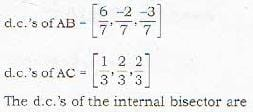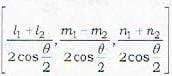where θ is the angle between AB (with d.c.'s [l1, m1, n1]) and AC (with d.c.’s [l2, m2, n2)
∴ the d.c.’s of the internal bisector are proprotional to l1+l2, m1+m2, n1+n2
or proportional to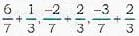or proportional to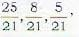or proportional to 25, 8, 5

Test: Dimensional Geometry - 4 - Question 14

Which of the following is incorrect?
If l1, m1, n1, : l2, m2, n2, : l3, m3, n3 be the direction cosines of three mutually perpendicular lines then

Detailed Solution for Test: Dimensional Geometry - 4 - Question 14

In fact, we shall have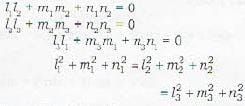Test: Dimensional Geometry - 4 - Question 15

The direction ratios of the line, which is equally inclined to the three mutually perpendicular lines with direction cosines l1, m1, n1: l2, m2, n2 : l3, m3, n3; are given by

Detailed Solution for Test: Dimensional Geometry - 4 - Question 15

Proof: Let the three mutually perpendicular lines be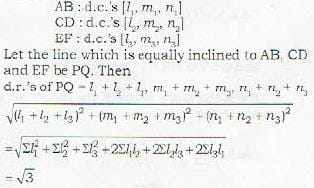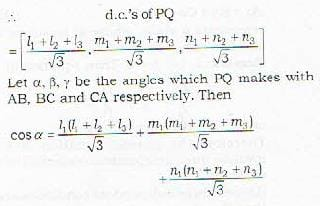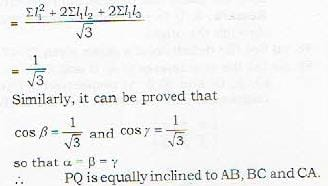Test: Dimensional Geometry - 4 - Question 16

“If O, A, B, C be the four points not lying in the same plane such that OA ⊥ BC, OB ⊥ CA, then OC ⊥ AB". if the points O, A, B, C are coplanar, then the above reduces to

Test: Dimensional Geometry - 4 - Question 17

If a1, b1, c1 and a2, b2, c2 are the direction ratios of two lines, then the angle between them is not given by

Test: Dimensional Geometry - 4 - Question 18

If the edges of a rectangular parallelepiped are OA , OB and OC along coordinate axes such that OA = a, OB = b, OC = c and PL, PM and PN are the perpendiculars drawn from P(a, b, c) on XY, ZX and YZ planes respectively, then angle between OP and AN is given by

Test: Dimensional Geometry - 4 - Question 19

If a variable line in two adjacent positions has direction cosines as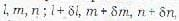then the small angle dθ between those two positions.in given by

Detailed Solution for Test: Dimensional Geometry - 4 - Question 19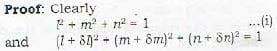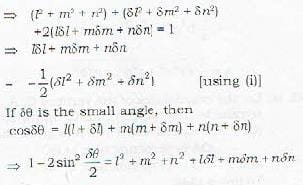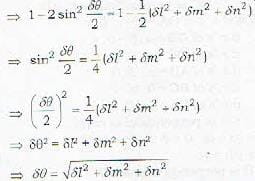Test: Dimensional Geometry - 4 - Question 20

The area of the triangle with vertices (0, 0, 0), (0, b, 0) and (0, 0, c) is given by

Detailed Solution for Test: Dimensional Geometry - 4 - Question 20

Proof: The area of the triangle OAB, where O is the origin and the coordinates of A and B are (x1, y1, z1) a n d [x2, y2, z2) respectively,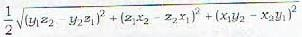Here since the coordinates of A and B are (0,b,0) and (0.0,c), therefore the required area A is given by

## Topic-wise Tests & Solved Examples for IIT JAM Mathematics

27 docs|150 tests
 Use Code STAYHOME200 and get INR 200 additional OFF Use Coupon Code
Information about Test: Dimensional Geometry - 4 Page
In this test you can find the Exam questions for Test: Dimensional Geometry - 4 solved & explained in the simplest way possible. Besides giving Questions and answers for Test: Dimensional Geometry - 4, EduRev gives you an ample number of Online tests for practice

## Topic-wise Tests & Solved Examples for IIT JAM Mathematics

27 docs|150 tests